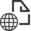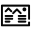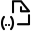ReleasedDataset

# GOCE gravity field model by means of the space-wise approach (release R5)

## Cite as:

Gatti, Andrea; Reguzzoni, Mirko (2017): GOCE gravity field model by means of the space-wise approach (release R5). GFZ Data Services. http://doi.org/10.5880/icgem.2017.005

## Status

I   N       R   E   V   I   E   W : Gatti, Andrea; Reguzzoni, Mirko (2017): GOCE gravity field model by means of the space-wise approach (release R5). GFZ Data Services. http://doi.org/10.5880/icgem.2017.005

##Abstract

GOCE input data:
- Orbits: SST_PRD_2I (reduced dynamic orbits for geo-locating gravity gradients); SST_PKI_2I (kinematic orbits for long-wavelength gravity field recovery); SST_PCV_2I (variance information of kinematic orbit positions); SST_PRM_2I (rotation between inertial and Earth-fixed reference frames)
- Attitude: EGG_IAQ_2C
- Non-conservative accelerations: EGG_CCD_2C
- Data period: 01/11/2009 - 20/10/2013

A-priori information used:
- No corrections to any prior gravity field model are computed (GOCE-only model).
- EIGEN-6C4 and GOCO05C are used for signal covariance modelling.
- FES2004 is used for ocean tide modelling.

Processing procedure:
- The space-wise approach is a multi-step collocation procedure, developed in the framework of the GOCE HPF data processing for the estimation of gravity gradient grids at satellite altitude. By analysing these grids, spherical harmonic coefficients of the Earth gravitational field and their error covariance matrix can be computed.
- SST model: gravitational potential estimation by energy conservation approach applied to kinematic orbits; grid interpolation of gravitational potential at mean satellite altitude by least-squares adjustment with local collocation refinement; spherical harmonic analysis of the estimated grids by numerical integration.
- SST+SGG model: orbital filtering of the data reduced by SST model (Wiener filter followed by whitening filter); grid interpolation of gravitational gradients at mean satellite altitude by local collocation; spherical harmonic analysis of the estimated grids by numerical integration.
- The full error covariance matrices of the estimated grids and spherical harmonic coefficients are derived by Monte Carlo simulations.

Remarks:
- The maximum spherical harmonic degree is 330 because this is the maximum degree used for the modelling of the signal covariance functions in the local collocation gridding.
- The spherical harmonic coefficients with the highest degrees have globally a small signal power, but they could contribute to better model local areas with a high signal-to-noise ratio.
- Any truncation of the spherical harmonic expansion to a maximum degree lower than 330 could introduce errors due to the correlation of the estimated spherical harmonic coefficients.
- The variance-covariance error information of the estimated spherical harmonic coefficients is computed by Monte Carlo simulations, also including the signal omission error up to degree and order 330.
- An error covariance propagation to functionals of the gravitational potential by only using coefficient error variances could be strongly approximated because coefficient error correlations are significant. The use of the full error variance-covariance matrix is therefore recommended.

## Parameters

 product_type gravity_field modelname GO_CONS_EGM_SPW_2I_20091101T055226_20131020T033415_0002 earth_gravity_constant 3.986004415E+14 [m3/s2] radius 6.378136460E+06 [m] max_degree 330 errors formal norm fully_normalized tide_system tide_free

## Contact

• Reguzzoni, Mirko; Department of Civil and Environmental Engineering (DICA), Politecnico di Milano, ITALY;

## Contributors

Migliaccio, Federica; Sansò, Fernando; Ince, Elmas Sinem; Reißland, Sven; Barthelmes, Franz

## Keywords

global gravitational model, ICGEM, GOCE, Geodesy

## Location

Click/hover over markers or bounding boxes to see related details. Click/hover over details to see related marker or bounding box.